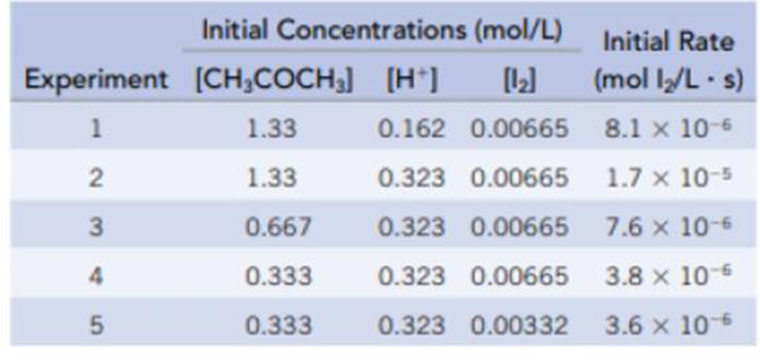# The acid-catalyzed iodination of acetone CH 3 COCH 3 (aq) + I 2 (aq) → CH 3 COCH 2 I(aq) + HI(aq) is a common laboratory experiment used in general chemistry courses to teach the method of initial rates. The reaction is followed spectrophotometrically by the disappearance of the color of iodine in the solution. The following data (J. P. Birk and D. L Walters, Journal of Chemical Education , Vol. 69, p. 585, 1992) were collected at 23 °C for this reaction. Determine the rate law for this reaction.### Chemistry & Chemical Reactivity

9th Edition
John C. Kotz + 3 others
Publisher: Cengage Learning
ISBN: 9781133949640

#### Solutions

Chapter
Section### Chemistry & Chemical Reactivity

9th Edition
John C. Kotz + 3 others
Publisher: Cengage Learning
ISBN: 9781133949640
Chapter 14, Problem 86IL
Textbook Problem
93 views

## The acid-catalyzed iodination of acetoneCH3COCH3(aq) + I2(aq) → CH3COCH2I(aq) + HI(aq)is a common laboratory experiment used in general chemistry courses to teach the method of initial rates. The reaction is followed spectrophotometrically by the disappearance of the color of iodine in the solution. The following data (J. P. Birk and D. L Walters, Journal of Chemical Education, Vol. 69, p. 585, 1992) were collected at 23 °C for this reaction.Determine the rate law for this reaction.

Interpretation Introduction

Interpretation:

The rate law should be determined for the given chemical reaction.

Concept Introduction:

Rate law: It is generally the rate equation that consists of the reaction rate with the concentration or the pressures of the reactants and constant parameters.

Rate constant: The rate constant for a chemical reaction is the proportionality term in the chemical reaction rate law which gives the relationship between the rate and the concentration of the reactant present in the chemical reaction.

### Explanation of Solution

The rate law is obtained by first determining the order of each reactant present in the given reaction. The order of each reactant is obtained by using the given set of concentration and the rate data as follows,

The order for CH3COCH3 is obtained by considering the experiment 2 and 3 from the given data.

23isasfollows,[CH3COCH3]2[CH3COCH3]3[H+]2[H+]3[I2]2[I2]3=[Rate]2[Rate]31.330.3230.006651.7×10-50.6670.3230.006657.6×10-6 _2m21

The order for CH3COCH3 is 1.

Similarly, the order for H+ is obtained as follow,

12isasfollows,[CH3COCH3]1[CH3COCH3]2[H+]1[H+]2[I2]1[I2]2=[Rate]1[Rate]21.330.1620.006658.1×10-61.330.3230.006651

### Still sussing out bartleby?

Check out a sample textbook solution.

See a sample solution

#### The Solution to Your Study Problems

Bartleby provides explanations to thousands of textbook problems written by our experts, many with advanced degrees!

Get Started

Find more solutions based on key concepts
How do eating disorders affect health?

Understanding Nutrition (MindTap Course List)

Calorie deficit is no longer the primary strategy for weight management.

Nutrition: Concepts and Controversies - Standalone book (MindTap Course List)

What impact has recombinant DNA technology had on genetics and society?

Human Heredity: Principles and Issues (MindTap Course List)

Review. (a) Show that a magnetic dipole in a uniform magnetic field, displaced from its equilibrium orientation...

Physics for Scientists and Engineers, Technology Update (No access codes included)